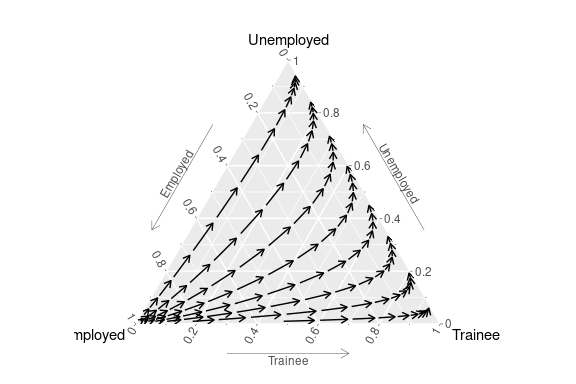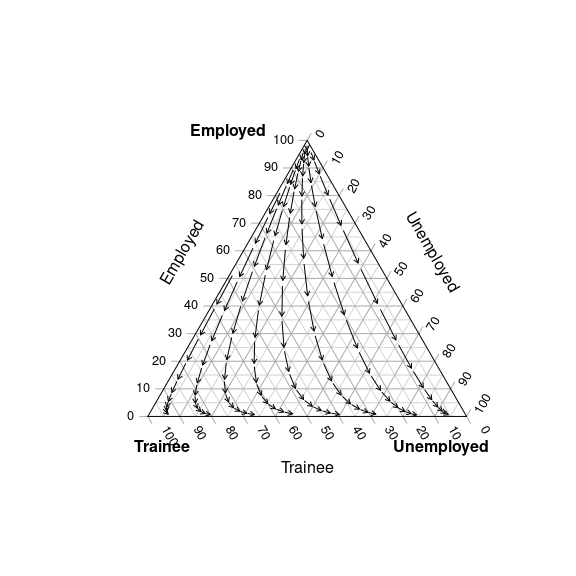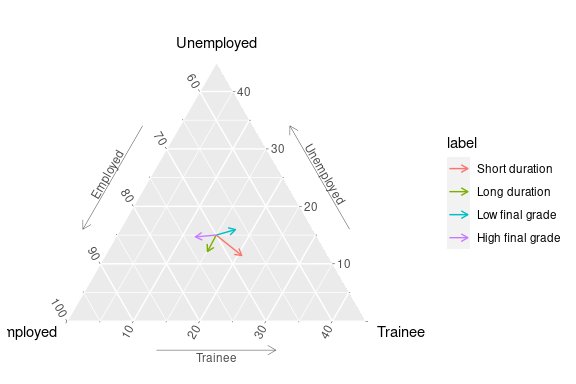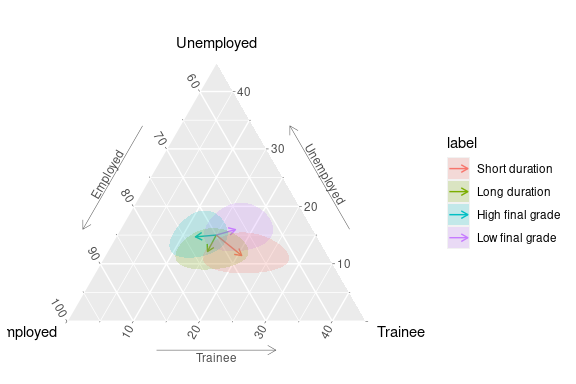# An overview of package plot3logit

## Introduction

The package permits the covariate effects of trinomial regression models to be represented graphically by means of a ternary plot. The aim of the plots is helping the interpretation of regression coefficients in terms of the effects that a change in regressors’ values has on the probability distribution of the dependent variable. Such changes may involve either a single regressor, or a group of them (composite changes), and the package permits both cases to be handled in a user-friendly way. Methodological details are illustrated and discussed in Santi, Dickson, and Espa (2019).

The package can read the results of both categorical and ordinal trinomial logit regression fitted by various functions (see the next section) and creates a field3logit object which may be represented by means of functions gg3logit and stat_field3logit.

The plot3logit package inherits graphical classes and methods from the package ggtern (Hamilton and Ferry 2018) which, in turn, is based on the package ggplot2 (Wickham 2016).

Graphical representation based on standard graphics is made available through the package Ternary (Smith 2017) by functions plot3logit and TernaryField, and by the plot method of field3logit objects.

See the help of field3logit for representing composite effects and multifield3logit for drawing multiple fields.

## Compatibility

Function field3logit of package plot3logit can read trinomial regression estimates from the output of the following functions:

• polr of package MASS (ordinal logit regression);
• mlogit of package mlogit (logit regression);
• multinom of package nnet (logit regression);
• clm and clm2 of package ordinal (ordinal logit regression);
• vgam and vglm of package VGAM (logit regression).

Explicit estimates can be passed to field3logit() by means of a named list too (see the help of field3logit); moreover, users can easily extend the range of objects that can be processed by field3logit() by implementing the S3 methods of the generic extract3logit (see the help).

In the following, an example is provided for each object field3logit can work with. First, however, package plot3logit must be attached and dataset cross_1year must be loaded:

library(plot3logit)
data(cross_1year)
str(cross_1year)
#> 'data.frame':    3282 obs. of  7 variables:
#>  $employment_sit: Factor w/ 3 levels "Employed","Unemployed",..: 1 1 1 3 1 1 1 1 1 2 ... #>$ gender        : Factor w/ 2 levels "Male","Female": 2 1 2 2 2 1 2 1 1 2 ...
#>  $finalgrade : Factor w/ 3 levels "Average","Low",..: 3 3 1 1 2 3 1 1 2 3 ... #>$ duration      : Factor w/ 3 levels "Average","Short",..: 3 3 3 3 3 3 1 3 3 3 ...
#>  $social_class : Factor w/ 5 levels "Working class",..: 1 2 4 4 3 3 1 4 1 2 ... #>$ irregularity  : Factor w/ 3 levels "Average","Low",..: 1 1 3 3 3 3 1 3 3 3 ...
x = mydata$finalgrade, levels = c('Low', 'Average', 'High'), ordered = TRUE ) mod0 <- polr(finalgrade ~ gender + irregularity, data = mydata) field3logit(mod0, 'genderFemale') ### Package mlogit Package mlogit of package mlogit (Croissant 2020) can fit a wide range of models. The current implementation of plot3logit only works with pure trinomial models, where only specific coefficients are considered: library(mlogit) mydata <- mlogit.data(cross_1year, choice = 'employment_sit', shape = 'wide') mod0 <- mlogit(employment_sit ~ 0 | gender + finalgrade, data = mydata) field3logit(mod0, 'genderFemale') ### Package nnet Function multinom of package nnet (Venables and Ripley 2002) fits a multinomial model by means of a very simple syntax: library(nnet) mod0 <- multinom(employment_sit ~ gender + finalgrade, data = cross_1year) field3logit(mod0, 'genderFemale') ### Package ordinal Package ordinal (Christensen 2019) permits ordinal multinomial logistic models to be fitted by means of two functions: clm and clm2. The latter is “a new improved implementation” of the former (see the help of clm2), in both cases the syntax is simple and standard. Unlike polr of package MASS (Venables and Ripley 2002), the dependent variable may be an unordered factor, and in that case both clm and clm2 consider the vector of the labels as ordered: if this is not the case, the dependent variable should be properly redefined. library(ordinal) mydata$finalgrade <- factor(mydata\$finalgrade, c('Low', 'Average', 'High'))

mod0 <- clm(finalgrade ~ gender + irregularity, data = mydata)
field3logit(mod0, 'genderFemale')

mod0 <- clm2(finalgrade ~ gender + irregularity, data = mydata)
field3logit(mod0, 'genderFemale')

### Package VGAM

Package VGAM (Yee 2010) permits multinomial logistic models to be fitted by means of two functions: vgam and vglm. In case of multinomial logistic models they share the same syntax:

library(VGAM)

mod0 <- vgam(
formula = employment_sit ~ gender + finalgrade,
family = multinomial(),
data = cross_1year
)
field3logit(mod0, 'genderFemale')

mod0 <- vglm(
formula = employment_sit ~ gender + finalgrade,
family = multinomial(),
data = cross_1year
)
field3logit(mod0, 'genderFemale')

### Reading from list

Point estimates and other information on a fitted model may be passed as a named list to field3logit. In case of a non-ordinal trinomial model, the matrix of coefficients (component B) should be a matrix with named rows and two columns, whereas possible levels of the dependent variable should be included as a separate component (levels) which should be a character vector of length three, whose first component is the reference level of the model (see the help of extract3logit for details):

mod0 <- list(
B = matrix(
data = c(-2.05, 0.46, -2.46, 0.37, -1.2, 0.8, 0.7, 0.4),
ncol = 2,
dimnames = list(c('Intercept', 'X1', 'X2', 'X3'))
),
levels = c('Class A', 'Class B', 'Class C')
)
field3logit(mod0, 'X1')

## A brief example

Fit a trilogit model by means of package nnet where the student’s employment situation is analysed with respect to all variables in the dataset cross_1year:

library(plot3logit)
data(cross_1year)
library(nnet)
mod0 <- multinom(employment_sit ~ ., data = cross_1year)
#> # weights:  42 (26 variable)
#> initial  value 3605.645531
#> iter  10 value 2167.042903
#> iter  20 value 2136.782685
#> iter  30 value 2134.363158
#> final  value 2134.352162
#> converged

The gender effect is analysed by means of a ternary plot which is generated in two steps.

Firstly, the vector field is computed:

field0 <- field3logit(mod0, 'genderFemale')

Secondly, the field is represented on a ternary plot, using either gg-graphics:

gg3logit(field0) + stat_field3logit()or standard graphics:

plot(field0)## How to specify the covariate changes

Ternary plots represent the effect of a change in covariate values on the probability distribution of the dependent variable. The function field3logit permits such change to be specified in three different ways: explicitly, as a numeric vector, or implicitly, either by means of a named numeric vector, or by means of an R expressions. All three methods are briefly illustrated below.

As an example, the following subsections refer to this trinomial logistic regression model:

library(plot3logit)
library(nnet)
mod0 <- multinom(employment_sit ~ finalgrade + irregularity + hsscore, cross_1year)
#> # weights:  21 (12 variable)
#> initial  value 3605.645531
#> iter  10 value 2187.709284
#> iter  20 value 2157.087955
#> final  value 2157.087854
#> converged
mod0
#> Call:
#> multinom(formula = employment_sit ~ finalgrade + irregularity +
#>     hsscore, data = cross_1year)
#>
#> Coefficients:
#> Unemployed  -0.4481761    0.05551765    -0.07810893     -0.01874354
#> Trainee     -1.3751140    0.14456683    -0.26849829      0.05764144
#>            irregularityHigh      hsscore
#> Unemployed       0.15691595 -0.016619227
#> Trainee         -0.03477569 -0.009964381
#>
#> Residual Deviance: 4314.176
#> AIC: 4338.176

### Explicit specification of $$\Delta x$$

This method for specifying the change in the covariate values requires the vector $$\Delta x$$ to be explicitly defined, thus it may be suitable when $$\Delta x$$ results from some calculations. On the other hand, it is less user-friendly than implicit syntax, as it depends on the order of regressors in the design matrix.

#### First example

If the effect of a high final grade has to be assessed, the vector of changes $$\Delta x$$ can be set according to the position of the dummy variable finalgradeHigh in the matrix of coefficients of the model mod0:

coef(mod0)
#> Unemployed  -0.4481761    0.05551765    -0.07810893     -0.01874354
#> Trainee     -1.3751140    0.14456683    -0.26849829      0.05764144
#>            irregularityHigh      hsscore
#> Unemployed       0.15691595 -0.016619227
#> Trainee         -0.03477569 -0.009964381

in this case, we have that $\Delta x=[0, 0, 1, 0, 0, 0]'$ since finalgradeHigh is the fourth coefficient (including the intercept) of the matrix of coefficients.

It follows that the function field3logit can be invoked as it follows:

field3logit(mod0, c(0, 0, 1, 0, 0, 0))
#>  Object of class "field3logit"
#> -------------------------------
#> Label                    : <empty>
#> Possible outcomes        : Employed; Unemployed; Trainee
#> Reference level          : Employed
#> Type of model            : categorical
#> Effect                   : 0 0 1 0 0 0
#> Model has been read from : nnet::multinom
#> Number of stream lines   : 8
#> Number of arrows         : 182
#> Covariance matrix        : available
#> Confidence regions       : not available

#### Second example

It is also possible to set $$\Delta x$$ so as to consider changes involving more than one regressor, as well as fractional changes. In such cases, $$\Delta x$$ will consist in a vector where there are several non-zero elements which may take any positive or negative value.

Assume, for example, that we want to study the effect of a decrease by 10 in the high school final score, associated to an high final grade. In such a case, we have that: $\Delta x =[0, 0, 1, 0, 0, -10]'\,,$ thus:

field3logit(mod0, c(0, 0, 1, 0, 0, -10))
#>  Object of class "field3logit"
#> -------------------------------
#> Label                    : <empty>
#> Possible outcomes        : Employed; Unemployed; Trainee
#> Reference level          : Employed
#> Type of model            : categorical
#> Effect                   : 0 0 1 0 0 -10
#> Model has been read from : nnet::multinom
#> Number of stream lines   : 8
#> Number of arrows         : 166
#> Covariance matrix        : available
#> Confidence regions       : not available

### Implicit specifications of $$\Delta x$$

Unlike the explicit method, the implicit syntaxes allows the user to initialise the vector $$\Delta x$$ by specifying only the covariates which should vary. There are two possible syntaxes: one is based on named numeric vectors, the other is based on R code. In the following, both of them are illustrated.

#### First example

If the effect of a high final grade has to be assessed, the implicit syntaxes which allow to assess the effect of a unitary change of finalgradeHigh are the following:

# Named numeric vectors:
#>  Object of class "field3logit"
#> -------------------------------
#> Label                    : <empty>
#> Possible outcomes        : Employed; Unemployed; Trainee
#> Reference level          : Employed
#> Type of model            : categorical
#> Explicit effect          : 0 0 1 0 0 0
#> Model has been read from : nnet::multinom
#> Number of stream lines   : 8
#> Number of arrows         : 182
#> Covariance matrix        : available
#> Confidence regions       : not available

# R code:
#>  Object of class "field3logit"
#> -------------------------------
#> Label                    : <empty>
#> Possible outcomes        : Employed; Unemployed; Trainee
#> Reference level          : Employed
#> Type of model            : categorical
#> Explicit effect          : 0 0 1 0 0 0
#> Model has been read from : nnet::multinom
#> Number of stream lines   : 8
#> Number of arrows         : 182
#> Covariance matrix        : available
#> Confidence regions       : not available

Note that the console output produced by printing the output of field3logit shows both the implicit effect (line Effect) and the associated vector $$\Delta x$$ (line Explicit effect).

#### Second example

If we want to study the effect of a decrease by 10 in the high school final score, associated to an high final grade, the implicit syntaxes are:

# Named numeric vectors:
field3logit(mod0, c(finalgradeHigh = 1, hsscore = -10))
#>  Object of class "field3logit"
#> -------------------------------
#> Label                    : <empty>
#> Possible outcomes        : Employed; Unemployed; Trainee
#> Reference level          : Employed
#> Type of model            : categorical
#> Effect                   : finalgradeHigh - 10 * hsscore
#> Explicit effect          : 0 0 1 0 0 -10
#> Model has been read from : nnet::multinom
#> Number of stream lines   : 8
#> Number of arrows         : 166
#> Covariance matrix        : available
#> Confidence regions       : not available

# R code:
field3logit(mod0, 'finalgradeHigh - 10 * hsscore')
#>  Object of class "field3logit"
#> -------------------------------
#> Label                    : <empty>
#> Possible outcomes        : Employed; Unemployed; Trainee
#> Reference level          : Employed
#> Type of model            : categorical
#> Effect                   : finalgradeHigh - 10 * hsscore
#> Explicit effect          : 0 0 1 0 0 -10
#> Model has been read from : nnet::multinom
#> Number of stream lines   : 8
#> Number of arrows         : 166
#> Covariance matrix        : available
#> Confidence regions       : not available

Compare the line Explicit effect of this output to the line Effect of the same example in the previous section: as expected, they are the same.

## Comparing multiple effects

When effects of multiple changes have to be compared at a time, multiple fields should be computed and represented on the same plot. This task can be easily done by creating a multifield3logit object and directly representing it.

Since objects multifield3logit result by putting together two or more field3logit objects, the package plot3logit allows the user to create a multifield3logit object by adding up two or more filed3logit or multifield3logit objects using standard sum operator +.

Here it is an example. The following command fit a trilogit model where all available variables are used as regressors. Then four fields3logit objects are computed for assessing the effects of a some combined changes in the duration of studies and in students’ final degree score.

Note that each field is computed just with respect to a single probability distribution (refpoint) of the dependent variable, and only one arrow is computed. The reason of this is that we have to represent four fields on the same plot, thus olny a small number of arrows can be drawn in order to preserve the clarity of the graph.

data(cross_1year)
mod0 <- nnet::multinom(employment_sit ~ ., data = cross_1year)

refpoint <- list(c(0.7, 0.15, 0.15))

field_Sdur <- field3logit(mod0, 'durationShort', label = 'Short duration', p0 = refpoint, narrows = 1)
field_Ldur <- field3logit(mod0, 'durationLong', label = 'Long duration', p0 = refpoint, narrows = 1)
field_Hfgr <- field3logit(mod0, 'finalgradeHigh', label = 'High final grade', p0 = refpoint, narrows = 1)
field_Lfgr <- field3logit(mod0, 'finalgradeLow', label = 'Low final grade', p0 = refpoint, narrows = 1)

Now the multifield3logit object can be created by adding all the field3logit objects up together:

mfields <- field_Sdur + field_Ldur + field_Lfgr + field_Hfgr
mfields
#>  Object of class "multifield3logit"
#> ------------------------------------
#> Number of fields         : 4
#> Labels
#>   1. Short duration    (dX: durationShort)
#>   2. Long duration     (dX: durationLong)
#>   4. High final grade  (dX: finalgradeHigh)

and the multifield3logit object mfield can be represented in a graph:

gg3logit(mfields) +
stat_field3logit(aes(colour = label)) +
theme_zoom_L(0.45)The code needed for generating the object mfields may be conveniently made shorter in this way (see the help of field3logit for details on syntax):

depo <- list(
list(delta = 'durationShort', label = 'Short duration'),
list(delta = 'durationLong', label = 'Long duration'),
)

mfields <- field3logit(mod0, delta = depo, p0 = refpoint, narrows = 1)
mfields
#>  Object of class "multifield3logit"
#> ------------------------------------
#> Number of fields         : 4
#> Labels
#>   1. Short duration    (dX: durationShort)
#>   2. Long duration     (dX: durationLong)
#>   4. Low final grade   (dX: finalgradeLow)

It is also possible to rely on syntax based on delimiters <<...>> (see the help of field3logit, section Details) in order to create a multifield3logit object consisting of fields based on the effect of each dummy variable generated by the same factor regressor:

field3logit(mod0, delta = '<<finalgrade>>', p0 = refpoint, narrows = 1)
#>  Object of class "multifield3logit"
#> ------------------------------------
#> Number of fields         : 2
#> Labels
#>   1. Low   (dX: finalgradeLow)
#>   2. High  (dX: finalgradeHigh)

## Confidence regions

The package plot3logit allows also to draw the confidence regions associated to each effect, both in case of field3logit and multifield3logit objects.

The confidence regions can be computed when the function field3logit is called by setting the argument conf. Otherwise, they can be added later through the function add_confregions as it follows:

field0 <- add_confregions(field0, conf = 0.95)
field0
#>  Object of class "field3logit"
#> -------------------------------
#> Label                    : <empty>
#> Possible outcomes        : Employed; Unemployed; Trainee
#> Reference level          : Employed
#> Type of model            : categorical
#> Effect                   : genderFemale
#> Explicit effect          : 0 1 0 0 0 0 0 0 0 0 0 0 0
#> Model has been read from : nnet::multinom
#> Number of stream lines   : 8
#> Number of arrows         : 111
#> Covariance matrix        : available
#> Confidence regions       : 95%

and through the same syntax in case of multifield3logit objects:

mfields <- add_confregions(mfields, conf = 0.95)

The statistic stat_conf3logit permits confidence regions to be drawn, if available:

gg3logit(field0) + stat_field3logit() + stat_conf3logit()

and

gg3logit(mfields) +
stat_field3logit(aes(colour = label)) +
stat_conf3logit(aes(fill = label)) +
theme_zoom_L(0.45)